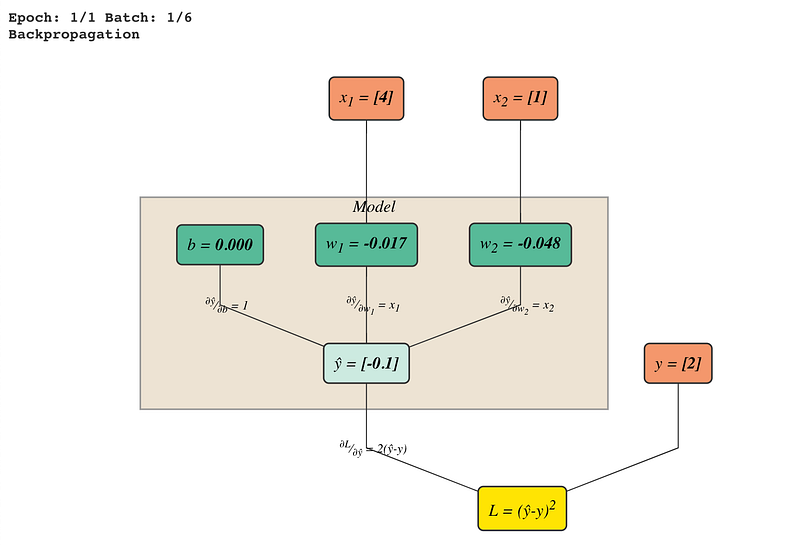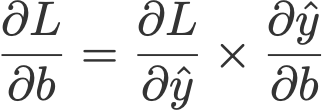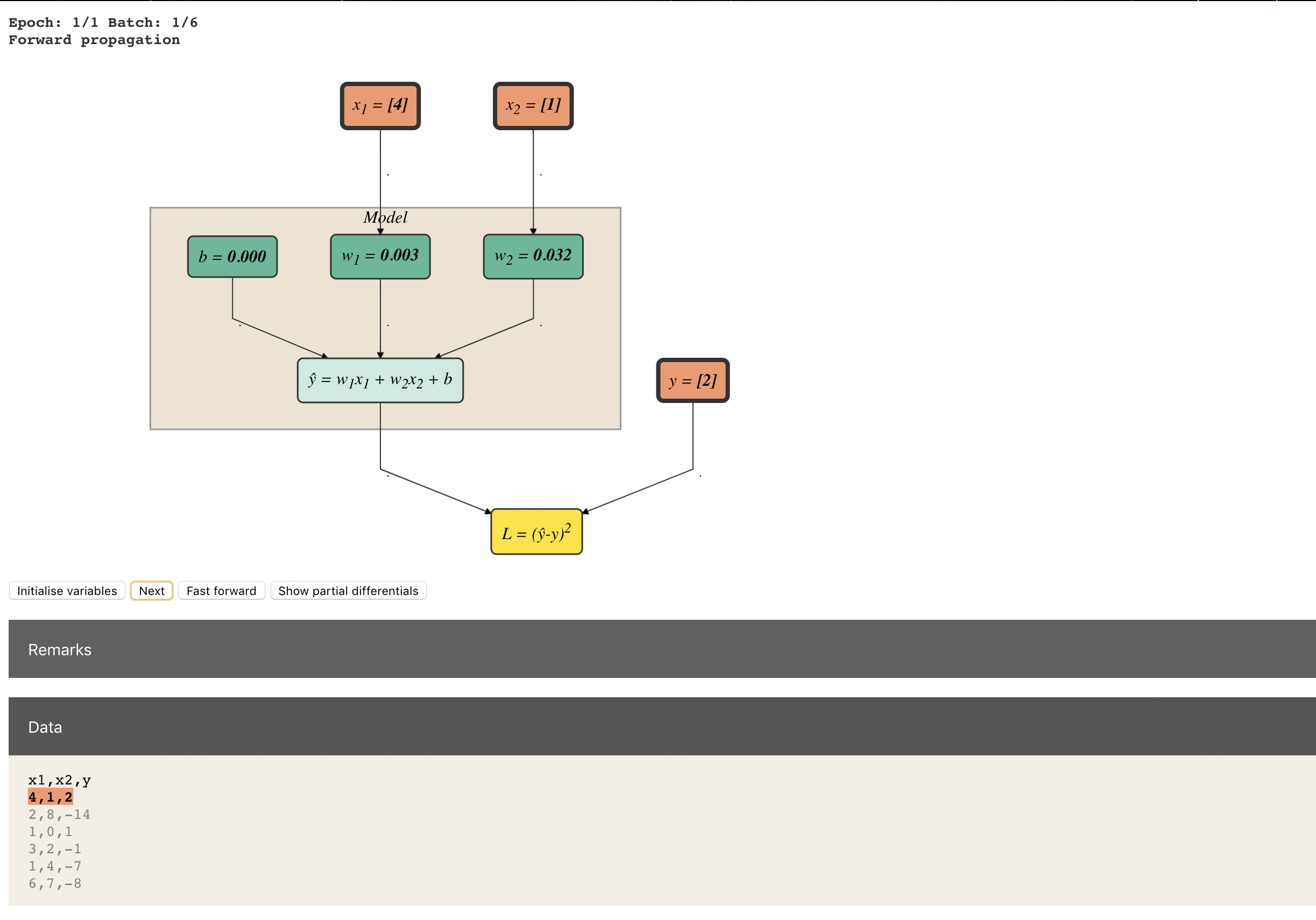# Tutorial: Linear Regression with Stochastic Gradient Descent

The JavaScript demo here. Photo by Lindsay Henwood on Unsplash.

This article should provide you a good start for us to dive deep into deep learning. Let me walk you through the step-by-step calculations for a linear regression task using stochastic gradient descent.

#### Contents

1. Preparation
1.1 Data
1.2 Model
1.3 Define loss function
1.4 Minimising loss function

2. Implementation
2.1 Forward propagation

2.1.1 Initialise weights (one-time)
2.1.2 Feed data
2.1.3 Compute ŷ
2.1.4 Compute loss
2.2 Backpropagation
2.2.1 Compute partial differentials
2.2.2 Update weights

### 1 Preparation

#### 1.1 Data

We have some data: as we observe the independent variables x₁ and x₂, we observe the dependent variable (or response variable) y along with it.

In our dataset, we have 6 examples (or observations).

  x1 x2  y
1) 4 1  2
2) 2 8 -14
3) 1 0  1
4) 3 2 -1
5) 1 4 -7
6) 6 7 -8

#### 1.2 Model

The next question to ask: “How are both x₁ and x₂ related to y?”

We believe that they are connected to each other by this equation:

Our job today is to find the ‘best’ and b values.

I have used the deep learning conventions w and b, which stand for weights and biases respectively. But note that linear regression is not deep learning.

#### 1.3 Define loss function

Let’s say at the end of this exercise, we’ve figured out our model to be

How do we know if our model is doing well?

We simply compare the predicted ŷ and the observed through a loss function.There are many ways to define the loss function but in this post, we define it as the squared difference between ŷ and y.

Generally, the smaller the L, the better.

#### 1.4 Minimise loss function

Because we want the difference between ŷ and to be small, we want to make an effort to minimise it. This is done through stochastic gradient descent optimisation. It is basically iteratively updating the values of w₁ and w₂ using the value of gradient, as in this equation:

This algorithm tries to find the right weights by constantly updating them, bearing in mind that we are seeking values that minimise the loss function.

You are w and you are on a graph (loss function). Your current value is w=5. You want to move to the lowest point in this graph (minimising the loss function).

You also know that, with your current value, your gradient is 2. You somehow must make use of this value to move on with life.

From high school math, 2 means you’re on an inclined slope and the only way you can descend is to move left, at this point.

If taking 5+2 means you’re going to the right climbing up the slope, then the only way is to take 5–2 which brings you to the left, descending down. So gradient descent is all about subtracting the value of the gradient from its current value.

### 2. Implementation

The workflow for training our model is simple: forward propagation (or feed-forward or forward pass), backpropagation.

Definition: training
Training just means regularly updating the values of your weights, put simply.

Below is the workflow.

— — — — — — — — — — — — —
2.1 Forward propagation
2.1.1 Initialise weights (one-time)
2.1.2 Feed data
2.1.3 Compute ŷ
2.1.4 Compute loss

2.2 Backpropagation
2.2.1 Compute partial differentials
2.2.2 Update weights
— — — — — — — — — — — — —

Let’s get started.

To keep track of all the values, we first build a ‘computation graph’ that comprises nodes colour-coded in

1. orange— the placeholders (x₁, x₂ and y),
2. dark green— the weights and bias (w₁, w₂ and b),
3. light green — the model (ŷ) connecting w₁, w₂, bx₁ and x₂, and
4. yellow — the loss function (L) connecting the ŷ and y.

For forward propagation, you should read this graph from top to bottom and for backpropagation bottom to top.

Note
I have adopted the term ‘placeholder’, a nomenclature used in
TensorFlow to refer to these ‘data variables’.
I will also use the term ‘weights’ to refer to
w and b collectively.

#### 2.1.1 Initialise weights (one-time)

Here we initialised the weights and bias as follows:

These are reflected in the dark green nodes in Fig. 2.1.1 below:

There are many ways to initialise weights (zeros, ones, uniform distribution, normal distribution, truncated normal distribution, etc.) but we won’t cover them in this post. In this tutorial, we initialised the weights by using truncated normal distribution and the bias with 0.

#### 2.1.2 Feed data

Next, we set the batch size to be 1 and we feed in this first batch of data.

Batch and batch size

We can divide our dataset into smaller groups of equal size. Each group is called a batch and consists of a specified number of examples, called batch size. If we multiply these two numbers, we should get back the number of observations in our data.

Here, our dataset consists of 6 examples and since we defined the batch size to be 1 in this training, we have 6 batches altogether.

Current batch of data used to feed in the model is bolded below:

   x1 x2  y1) 4 1  22) 2 8 -143) 1 0  14) 3 2 -15) 1 4 -76) 6 7 -8

In Fig. 2.1.2, the orange nodes are where we feed in the current batch of data.

Fig. 2.1.2: Feeding data to model with first batch (orange nodes)

#### 2.1.3 Compute ŷ

Now that we have the values of x₁, x₂, w₁, w₂ and b ready, let’s compute ŷ.

The value of ŷ (=-0.1) is reflected in the light green node below:

#### 2.1.4 Compute loss

How far is our predicted ŷ from the given y data? We compare them by calculating the loss function as defined earlier.

You can see this value in the yellow node in the computation graph.

It is a common practise to log the loss during training, together with other information like the epoch, batch and time taken. In my demo, you can see this under the Training progress panel.

#### 2.2.1 Compute partial differentials

Before we start adjusting the values of the weights and bias w₁, w₂ and b, let’s first compute all the partial differentials. These are needed later when we do the weight update.Fig. 2.2.1: Indicated partial differentials to the relevant edges on the graph

Namely, we compute all possible paths leading to every w and only, because these are the only variables which we are interested in updating. From Fig. 2.2.1 above, we see that there are 4 edges that we labeled with the partial differentials.

Recall the equations for the model and loss function:

The partial differentials are as follows:

(yellow) — ŷ (light green):

ŷ (light green) — (dark green):

ŷ (light green) — w₁ (dark green):

ŷ (light green) — w₂ (dark green):

Note that the values of the partial differentials follow the values from thecurrent batch. For example, in Eqn. 2.2.1C, x₁ = 4.

#### 2.2.2 Update weights

Observe the dark green nodes in Fig. 2.2.2 below. We see three things:
i) b changes from 0.000 to 0.212
ii) w₁ changes from -0.017 to 0.829
iii) w₂ changes from -0.048 to 0.164

Also pay attention to the ‘direction’ of the pathway from the yellow node to the green node. They go from bottom to top.

This is stochastic gradient descent — updating the weights using backpropagation, making use of the respective gradient values.

Let’s first focus on updating b. The formula for updating is

where

• b— current value
• b’— value after update
• η —learning rate, set to 0.05
• ∂L/∂b — gradient i.e. partial differential of L w.r.t. b

To get the gradient, we need to multiply the paths from L leading to b using chain rule:Eqn. 2.2.2B: Chain rule for partial differential of L w.r.t. b

We would require the current batch values of xy, ŷ and the partial differentials so let’s just place them below for easy reference:

Using the stochastic gradient descent equation in Eqn. 2.2.2A and plucking in all the values from Eqn. 2.2.2B-D gives us:

That’s it for updating b! Phew! We are left with updating w₁ and w₂, which we update in a similar fashion.

#### End of batch iteration

Congrats! That’s it for dealing with the first batch.

    x1 x2   y
1)   4  1   2  ✔
2)   2  8 -14
3)   1  0   1
4)   3  2  -1
5)   1  4  -7
6)   6  7  -8

Now we need to iterate the above-mentioned steps to the other 5 batches, namely examples 2 to 6.Iterating through batch 1 to 6 (apologies for the poor GIF quality! )

#### End of epoch

We complete 1 epoch when the model has iterated through all the batches once. In practise, we extend the epoch to more than 1.

One epoch is when our setup has seen all the observations in our dataset once. But one epoch is almost always never enough for the loss to converge. In practice, this number is manually tuned.

At the end of it all, you should get a final model, ready for inference, say:

Let’s have a review of the entire workflow in a pseudo-code:

initialise_weights()

for i in epochs:

for j in batches:

#forward propagation
feed_batch_data()
compute_ŷ()
compute_loss()

#backpropagation
compute_partial_differentials()
update_weights()

#### Improve training

One epoch is never enough for a stochastic gradient descent optimisation problems. Remember that in Fig. 4.1, our loss is at 4.48. If we increase the number of epochs, which means just increasing the number of times we update the weights and biases, we can converge it to a satisfactory low.

Below are the things you can improve the training:

• Extend training to more than 1 epoch
• Increase batch size
• Change optimiser (see my post on gradient descent optimisation algorithms here)
• Adjust learning rate (changing the learning rate value or using learning rate schedulers)
• Hold out a train-val-test set

I built an interactive explorable demo on linear regression with gradient descent in JavaScript. Here are the libraries I used:

• Dagre-D3 (GraphViz + d3.js) for rendering the graphs
• MathJax for rendering mathematical notations
• ApexCharts for plotting line charts
• jQuery

Check out the interactive demo here.

You might also like to check out A Line-by-Line Layman’s Guide to Linear Regression using TensorFlow, which focuses on coding linear regression using the TensorFlow library.

#### References

https://colah.github.io/posts/2015-08-Backprop/

#### Related Articles on Deep Learning

Animated RNN, LSTM and GRU

Line-by-Line Word2Vec Implementation (on word embeddings)

10 Gradient Descent Optimisation Algorithms + Cheat Sheet

Counting No. of Parameters in Deep Learning Models

Attn: Illustrated Attention

Thanks to Ren Jie and Derek for ideas, suggestions and corrections to this article.

Follow me on Twitter @remykarem for digested articles and demos on AI and Deep Learning.

## Author

•Raimi is an AI Engineer at AI Singapore. He likes building demos and write articles on machine learning.